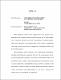## Convergence rate theory for global optimizationGlobal optimization is used to control complex systems whose response is an unknown function on a continuous domain. Response values can only be observed empirically by simulations, and cannot be accurately represented using closed-form mathematical expressions. Prediction of true optimizer in this context is usually accomplished by constructing a surrogate model that can be thought of as an interpolation of a discrete set of observed design points. This thesis includes study of convergence rates of epsilon-greedy global optimization under radial basis function interpolation. We derive both convergence rates and concentration inequalities for a general and widely used class of interpolation models known as radial basis functions, used in conjunction with a randomized algorithm that searches for solutions either within a small neighborhood of the current-best, or randomly over the entire domain. An interesting insight of this work is that the convergence rate is improved when the size of the local search region shrinks to zero over time in a certain way. My work precisely characterizes the rate of this shrinkage. Gaussian process regression is another tool that is widely used to construct surrogate models. A theoretical framework is developed for proving new moderate deviations inequalities on different types of error probabilities that arise in GP regression. Two specific examples of broad interest are the probability of falsely ordering pairs of points (incorrectly estimating one point as being better than another) and the tail probability of the estimation error of the minimum value. Our inequalities connect these probabilities to the mesh norm, which measures how well the design points fill the space. Convergence rates are further instantiated in settings of using a Gaussian kernel, and either deterministic or random design sequences. Convergence can be more rapid when we are not totally blind to the objective function. As an example, we present a work on simultaneous asymmetric orthogonal tensor decomposition. Tensor decomposition can be essentially viewed as a global optimization problem. However with the knowledge of the algebraic information from the observed tensor, the method only requires $O(\log(\log \frac{1}{\epsilon}))$ iterations to reach a precision of $\epsilon$.